### Cryptocurrency

Throughout this chapter, we've followed the development of DragonBucks (which was succeeded by CryptoniaCoin) and learned how certain mathematical functions can be used to secure a currency system.However, along the way we hinted at some potential security flaws with the Cryptonian system, and you may have picked up on some of them yourself.

In this exploration, we’ll point out some of the weaknesses of CryptoniaCoin and start to look at ways to resolve them. We won’t be able to patch all the security holes in this quiz, but we will address each of them by the end of the course.

# Cryptonian Vulnerabilities

One requirement of the CryptoniaCoin system is that we're able to represent everything as numbers. This means that we need a way to convert transaction notes into a unique ${\tt message}$ (which is also a number).

Consider a simple way for converting a transaction note to a number: concatenate the transaction amount, the public keys of the parties involved, and the date. For example, if we wanted to send $15$ coins from the account $({\tt pk: }5284)$ to account $4295$ on $10/17/2019,$ then $\tt message = 155284429510172019.$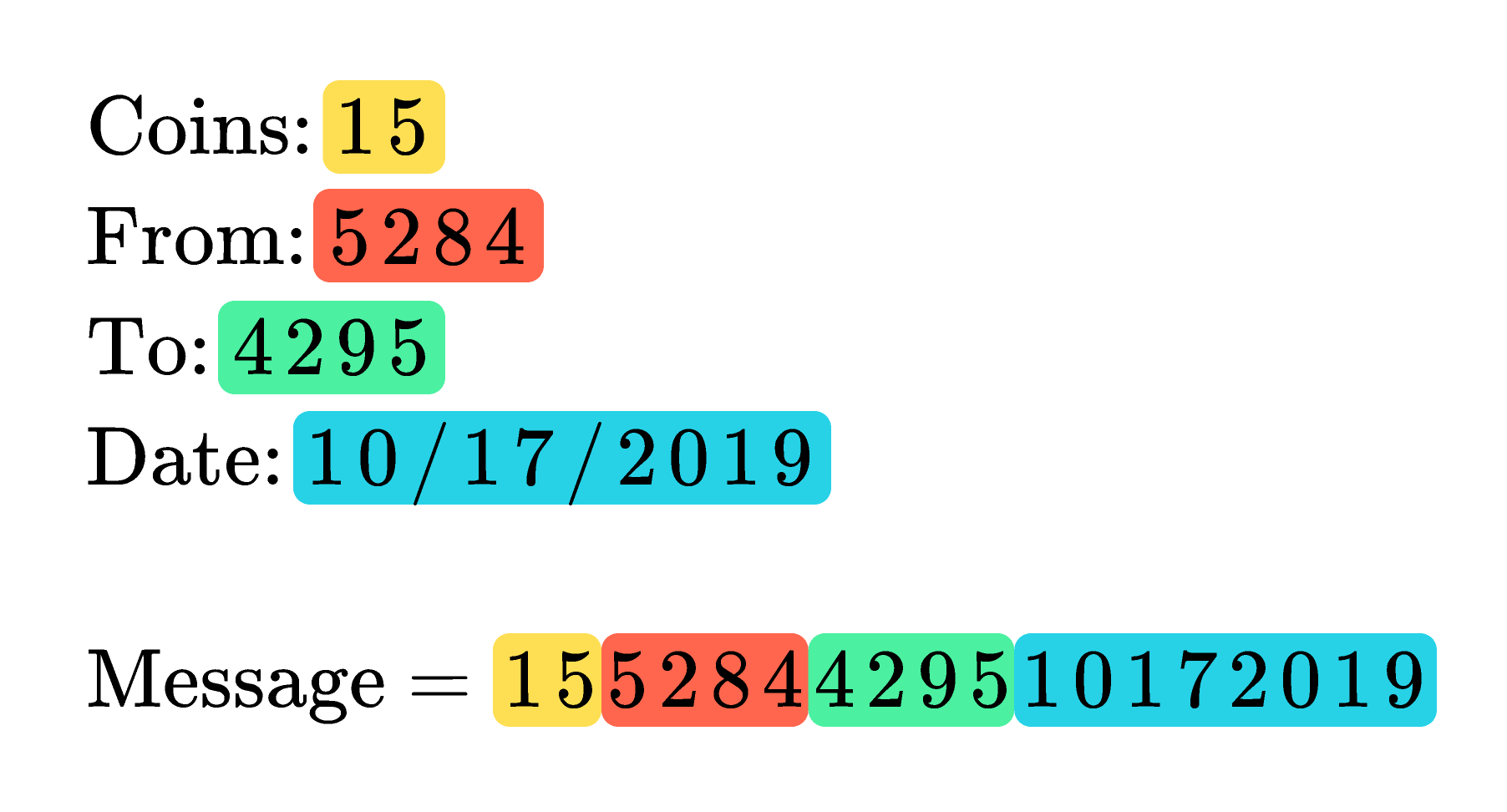What’s wrong with this method of converting a transaction note into a number?

Assume that all account numbers are the same number of digits and the date is always expressed with $8$ digits.

# Cryptonian Vulnerabilities

Suppose you wrote a note to buy a loaf of bread, but then realized you actually needed two loaves of bread, so you go back to the store and write another note to buy the second loaf.If the cost of each loaf is the same, you pay using the same account, and it’s on the same day, then using the system we proposed for the previous question, those two notes would be converted to the same $\tt message.$ This is problematic because the two identical messages would have the same signature, so either

1. you would have to disallow notes that are a copy of an existing note (which is inconvenient), or
2. someone could use unlimited versions of the same note once they have the valid signature of the first note (which is not secure).

# Cryptonian Vulnerabilities

Suppose we make a rule that notes that are identical to an existing note are automatically invalid. Then, if you tried to use two notes with the same $\tt message$ and the same signature, only the first would be honored.

If we want someone to be able to make two similar payments on the same day, we could try changing the way the $\tt message$ is constructed:

1. the $\tt message$ could include the precise time in addition to the date, or
2. the date could be replaced by a "transaction counter" associated with the sender's account that increments by $1$ for each new transaction.

Would either of these changes allow you to make two similar purchases on the same day?

# Cryptonian Vulnerabilities

For security, we never want a signature to be able to be used more than once, even for similar transactions. Fortunately, there are relatively easy fixes we can implement so every transaction results in different message numbers and therefore requires different signatures.

Different cryptocurrencies use different techniques to make this work in practice, and they’re all valid.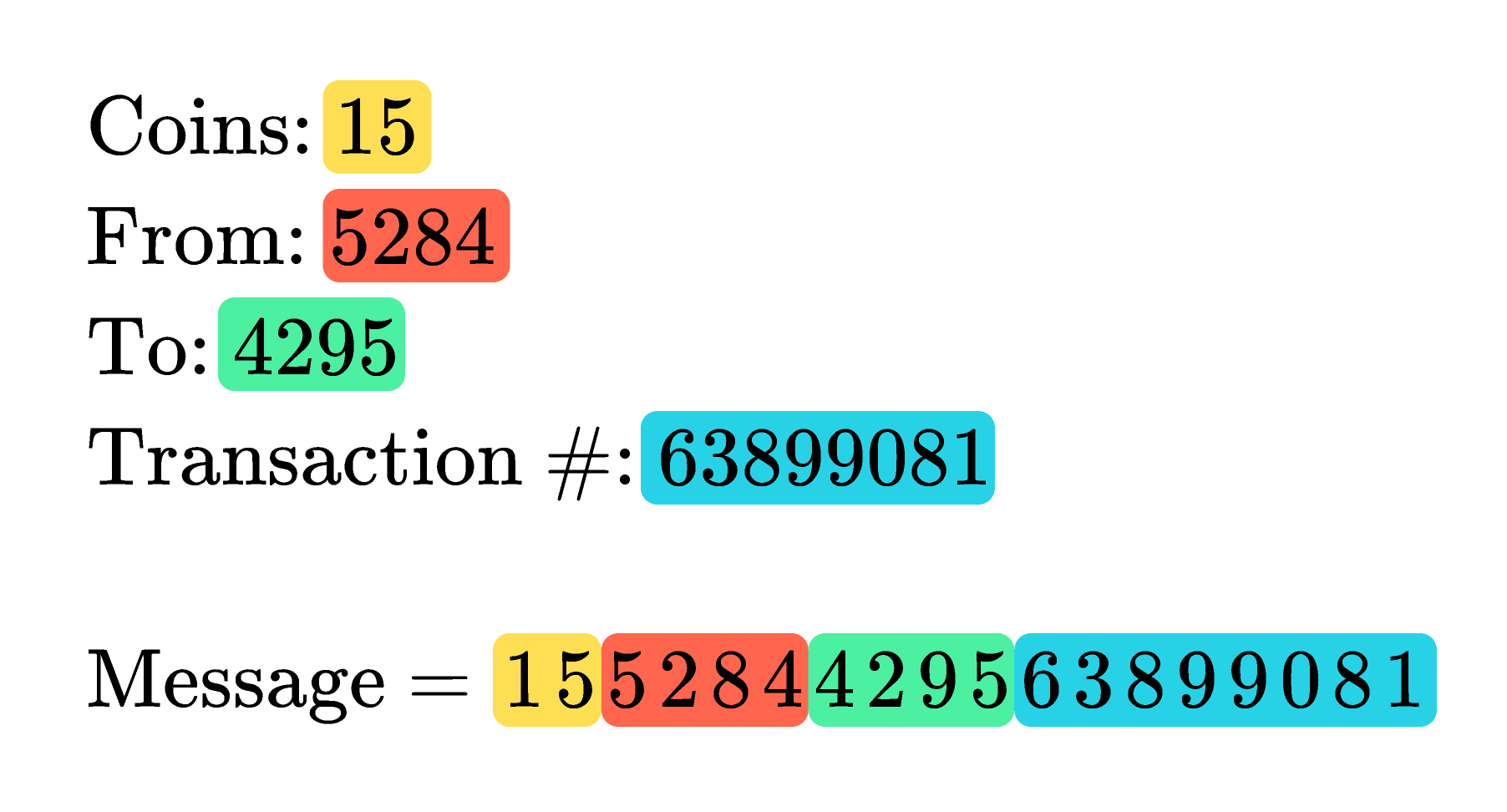Because the fixes are relatively easy, this doesn’t represent a major security concern for cryptocurrencies, but it's something we need to be conscious of when designing a verification scheme.

# Cryptonian Vulnerabilities

In DragonBucks, the dragon doesn’t need to know anyone’s secret key — the system we built allows anyone to verify signatures. Whether you’re in charge of updating the account tallies or not, you can verify a signature using public information.

However, if you don’t tell the dragon (or anyone else, for that matter) your secret key, then there's no one to ensure that it’s actually different from everyone else's.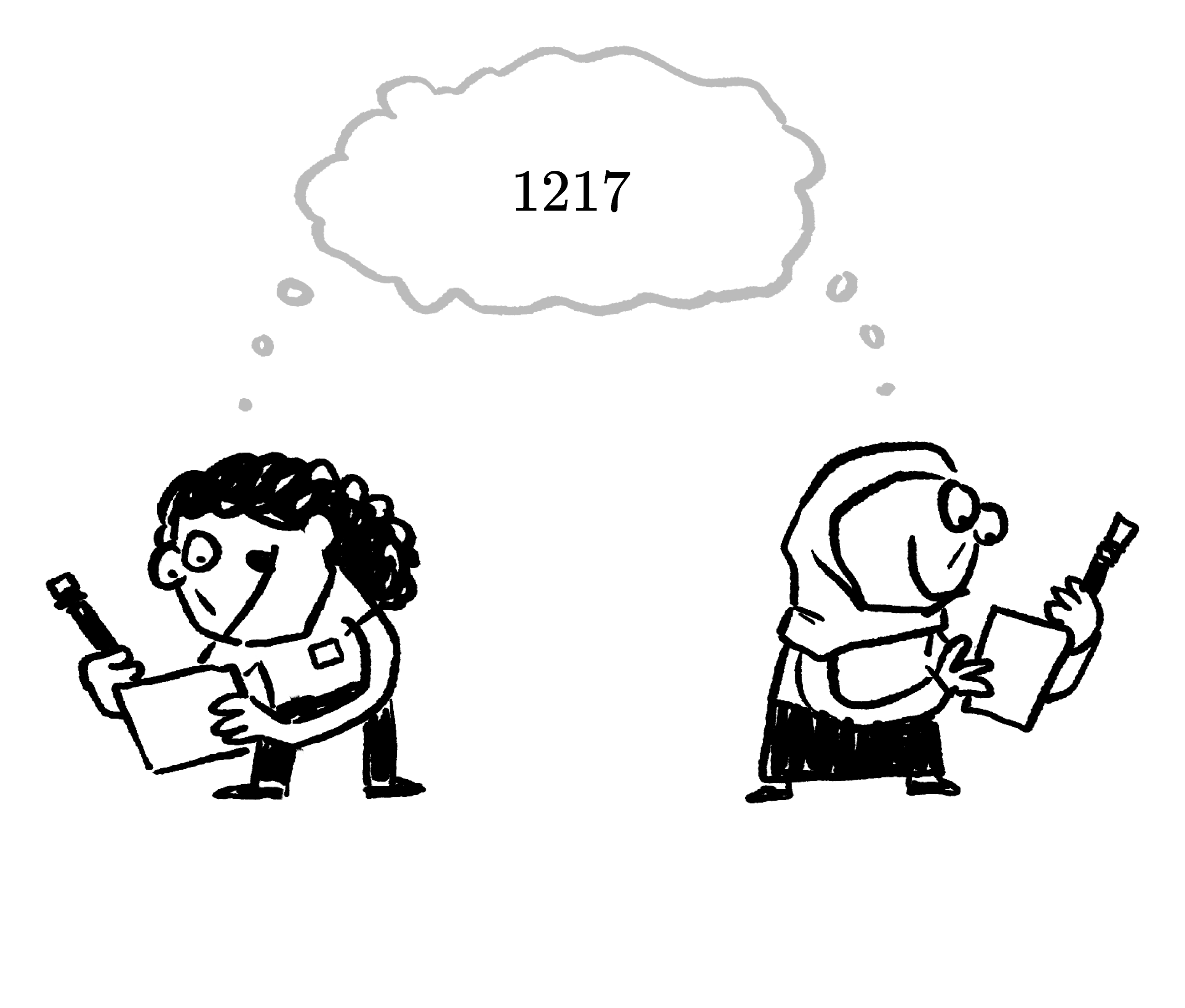If you did have the same secret key as someone else, it would be pretty obvious since you’d also have the same public key. But you could also have a different secret key from someone else, yet still have the same public key.

# Cryptonian Vulnerabilities

We can see this using the calculator below, which implements the modular arithmetic of the DragonBucks system.

Try using $n = 29, g = 17, s = 35,$ and an $m$ of your choosing. Next, use the same $n, g,$ and $m,$ but set the secret key $s = 64.$

With the sample values above, $s=35$ and $s=64$ should lead to the same public key. If you had the same public key as someone else but a different secret key, would your accounts be distinct?

# Cryptonian Vulnerabilities

Even if the modular arithmetic of our system protects someone else from being able to back-calculate your $\left(s \bmod{n}\right)$ from your $\tt publicKey$ or a $\tt signature$ you've issued, it's still possible for someone else to pick the same public key for themselves by chance.

If you have the same $\tt publicKey$ as someone else, that means that your secret keys are the same in $\text{mod }n,$ i.e. $s^\prime\bmod{n} = s \bmod{n}.$ Though you each have a distinct $\tt secretKey,$ for the purposes of the Cryptonian message-signing system, your accounts are practically the same. You would be able to sign valid messages on behalf of each other.

This represents a big security flaw — if you happen to choose the same secret key that someone else is already using, then you’d be able to spend all their money.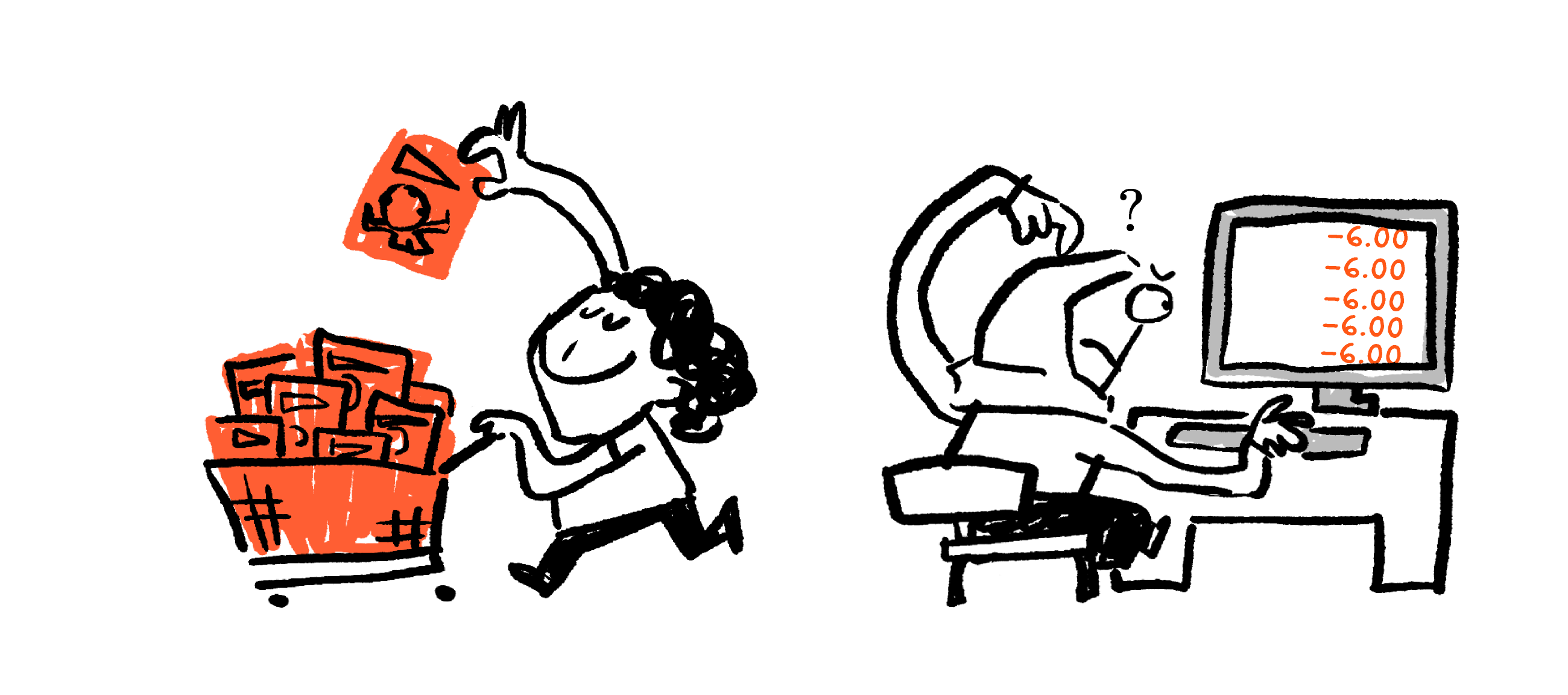What could we do to reduce this security risk (while keeping the rest of the system the same)?

# Cryptonian Vulnerabilities

Even if controlling the issuing of keys did work, it would require trust in a central authority that controlled those keys, representing a fundamentally different type of currency.

While it may seem strange, the best protection against someone else having the same public key as you is simply increasing the number of possible public keys. The more possible public keys there are, the less likely you are to find someone else’s (whether you’re trying to do it or not).

Just how many possibilities we'd need for the system to be considered "safe" will be explored later in this course. But to give you a preview, some real systems use secret keys long enough that it would take more than $\sim10^{50}$ random guesses before you could expect to replicate another user's key.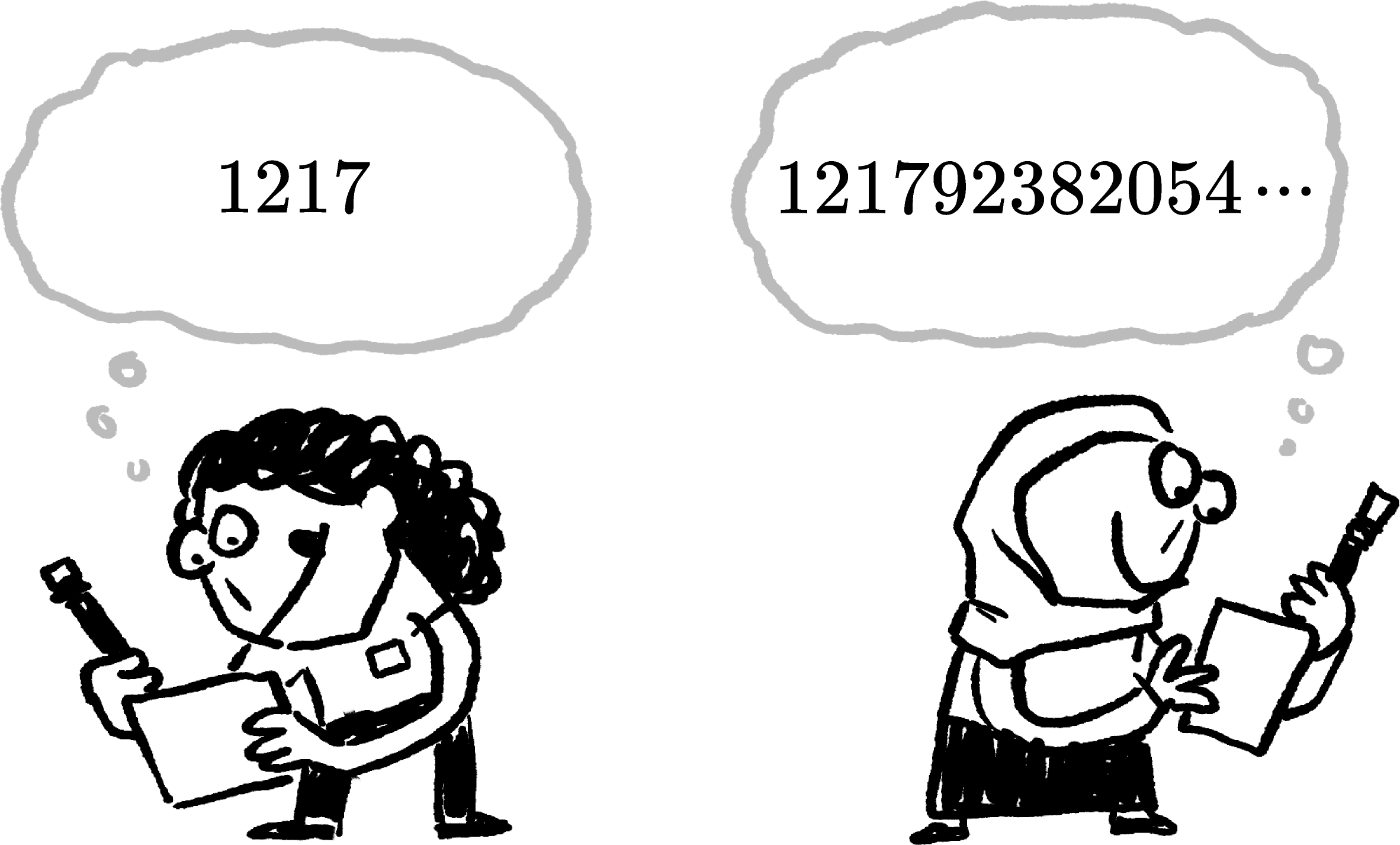# Cryptonian Vulnerabilities

We claimed the Cryptonian system is secure because you can share your public key, $(g\times s) \bmod{n},$ without revealing the value of $s \bmod{n}.$ Indeed, you can't invert (undo, in other words) modular multiplication by simply dividing by the original factor and applying $\bmod{},$ e.g. $\frac{(g\times s) \bmod{n}}{g} \stackrel{?}{=} s \bmod{n},$ but that doesn't mean it's impossible.

If we did have a way to "modularly divide" by $g,$ then $s \bmod{n}$ would not be safe. Let's take a closer look at what it would take to do so.

Suppose that, instead of dividing by $g,$ we multiply by another constant $k.$ The goal would be for $k$ to have the following effect: $\left(k\times \texttt{publicKey} \right) \bmod{n} = s \bmod{n}.$

What property would we need $k$ to have for this to work?

# Cryptonian Vulnerabilities

If we could find $k$ such that $\left(k\times g\right) \bmod{n} = 1,$ then it would allow us to "modularly divide" by $g$. We call the $k$ that satisfies this equation the "modular multiplicative inverse" of $g,$ and denote it $g^{-1}$ (not to be confused with the reciprocal of $g$ outside of modular arithmetic). So the $g^{-1}$ that lets us "divide" by $g$ must satisfy the equation:

$\left(g^{-1}\times g\right) \bmod{n} = 1.$

Even though we multiply $g^{-1},$ it’s effectively modular division since multiplying by $g^{-1}$ cancels $g$ from the expression in $\bmod{\>n}:$ \begin{aligned} g^{-1} \times \texttt{publicKey} &= \left(g^{-1}\times g \times s\right) \bmod{n} \\ &= (1 \times s) \bmod{n} \\ &= s \bmod{n}. \end{aligned} In effect, we use $g^{-1}$ to unlock $s\bmod{n}.$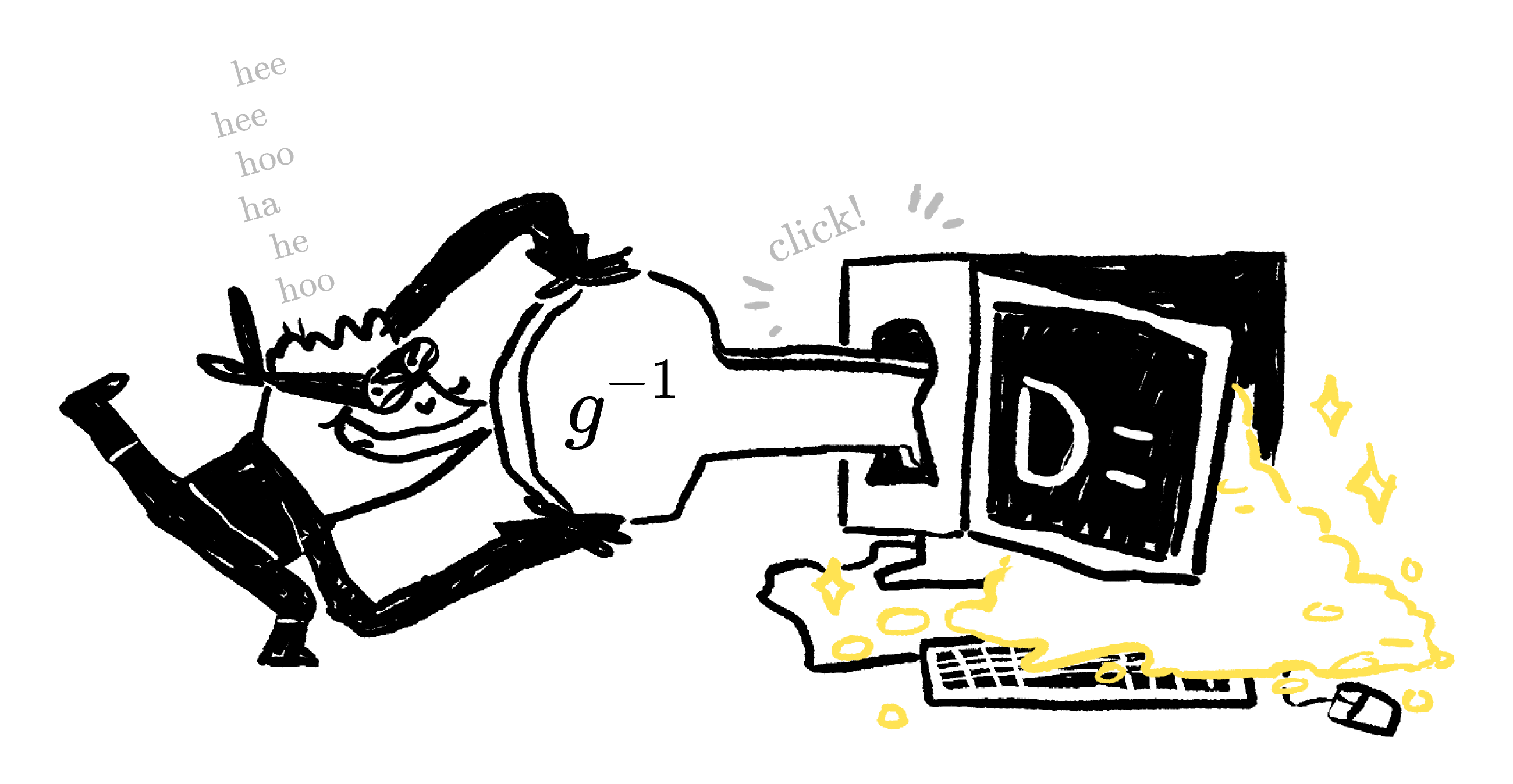For small $n$, we can find a $g^{-1}$ quickly with just guess and check.

Suppose the Cryptonians chose $g=5$ for their system. What is $5^{-1}$ in $\bmod{\>7}?$

You can use the modular calculator below to help to find the answer.

# Cryptonian Vulnerabilities

The existence of $g^{-1}$ is what fundamentally threatens the security of our modular multiplication verification scheme. For a small $n$ like the one we just looked at, you can find $g^{-1}$ through guess and check without taking too long and easily crack the system.

Making $n$ bigger will protect against this simple guess-and-check strategy, but as it turns out, there are more efficient ways to find $g^{-1}.$In the next chapter, we’ll see why this security hole can’t be plugged without changing some of the functions that make up our verification scheme, and what properties we need those functions to have in order to be sufficiently secure for a cryptocurrency.

See you there!

# Cryptonian Vulnerabilities

×